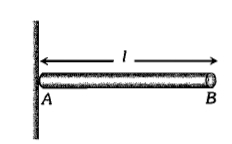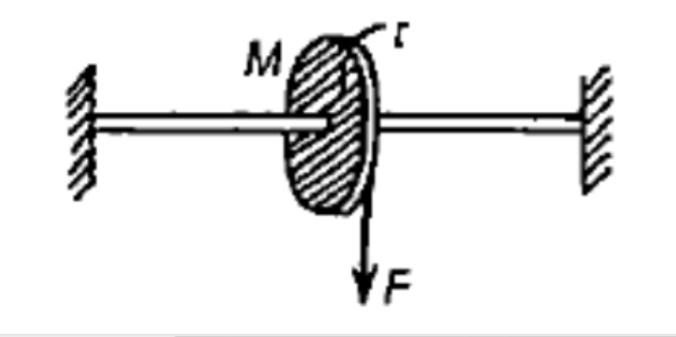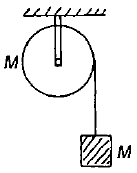A uniform rod AB of length l and mass m is free to rotate about point A. The rod is released from rest in horizontal position. Given that the moment of inerita of the rod about A is $\frac{{\mathrm{ml}}^{2}}{3}$ the initial angular acceleration of the rod will beConcept Questions :-

Torque
High Yielding Test Series + Question Bank - NEET 2020

Difficulty Level:

The magnitude of the impulse developed by a mass of 0.2 kg which changes its velocity from  is

1.  2.7 N-s

2.  1.8 N-s

3.  0.9 N-s

4.  3.6 N-s

Concept Questions :-

Linear momentum
High Yielding Test Series + Question Bank - NEET 2020

Difficulty Level:

A round disc of the moment of inertia ${\mathrm{l}}_{2}$ about its axis perpendicular to its plane and passing through its center is placed over another disc of the moment of inertial ${\mathrm{l}}_{1}$ rotating with an angular velocity $\omega$ about the same axis. The final angular velocity of the combination of discs is

1.

2.  $\mathrm{\omega }$

3.

4.

Concept Questions :-

Angular momentum
High Yielding Test Series + Question Bank - NEET 2020

Difficulty Level:

A light cord is wrapped around the rim of the wheel disc of radius r and a steady downward pull F is exerted on the cord, then the angular acceleration of the wheel is(1) $\frac{F}{Mr}$           (2) $\frac{2F}{M}$                 (3) $\frac{2F}{Mr}$                 (4) $\frac{M}{2F}$

High Yielding Test Series + Question Bank - NEET 2020

Difficulty Level:

A solid sphere is rotating in free space. If the radius of the sphere is increased keeping mass same which one of the following will not be affected
(1) Moment of inertia

(2) Angular momentum

(3) Angular velocity

(4) Rotational kinetic energy

Concept Questions :-

Angular momentum
High Yielding Test Series + Question Bank - NEET 2020

Difficulty Level:

A particle of mass 5 g is moving with a uniform speed of $3\sqrt{2}$cm/s in the x-y plane along the line y = $2\sqrt{5}$cm. The magnitude of its angular momentum about the origin in $\mathrm{g}-{\mathrm{cm}}^{2}/\mathrm{s}$ is

1.  0

2.  30

3.  $30\sqrt{2}$

4.  $30\sqrt{10}$

Concept Questions :-

Angular momentum
High Yielding Test Series + Question Bank - NEET 2020

Difficulty Level:

Three-point masses, each of mass m are placed at the corners of an equilateral triangle of side a. The moment of inertia of this system ab5ut an axis along one Side of the triangle is

1.

2.  ${\mathrm{ma}}^{2}$

3.  ${\mathrm{ma}}^{2}\frac{3}{4}$

4.  $\frac{3}{2}{\mathrm{ma}}^{2}$

Concept Questions :-

Moment of inertia
High Yielding Test Series + Question Bank - NEET 2020

Difficulty Level:

A mass M is supported by a massless string wound round a uniform cylinder of mass M and radius R. On releasing the mass from rest, it will fall with an acceleration1.  $\frac{\mathrm{g}}{3}$

2.  $\frac{\mathrm{g}}{2}$

3.  $\frac{2\mathrm{g}}{3}$

4.  g

Concept Questions :-

Torque
High Yielding Test Series + Question Bank - NEET 2020

Difficulty Level:

A closed tube partly filled with water lies in a horizontal plane. If the tube is rotated about perpendicular bisector, the moment of inertia of the system

(1) increases

(2) decreases

(3) remains constant

(4) depends on sense of rotation

Concept Questions :-

Moment of inertia
High Yielding Test Series + Question Bank - NEET 2020

Difficulty Level:

A disc is rotating With an angular speed of m. If a child sits on it, which of the following is conserved?

1.  kinetic energy

2.  potential energy

3.  linear momentum

4.  angular momentum

Concept Questions :-

Angular momentum